##### Open Access Journal of
###### eISSN: 2575-9086# ScienceResearch Article Volume 2 Issue 6

# Optimal long term operation policies associated with the generated affectations – low regulation capacity reservoirs

#### Sadoth Fabian Huerta Loera,,1 function clickButton(){ var name=document.getElementById('name').value; var descr=document.getElementById('descr').value; var unCopyslNo=document.getElementById('unCopyslNo').value; document.getElementById("mydiv").style.display = "none"; $.ajax({ type:"post", url:"https://medcraveonline.com/captchaCode/server_action", data: { 'name' :name, 'descr' :descr, 'unCopyslNo': unCopyslNo }, cache:false, success: function (html) { //alert('Data Send');$('#msg').html(html); } }); return false; } Verify Captcha Regret for the inconvenience: we are taking measures to prevent fraudulent form submissions by extractors and page crawlers. Please type the correct Captcha word to see email ID.function refreshPage(){ $("#mydiv").load(location.href + " #mydiv"); }$(document).ready(function () { //Disable cut copy paste $('#msg').bind('cut copy paste', function (e) { e.preventDefault(); }); //Disable mouse right click$("#msg").on("contextmenu",function(e){ return false; }); }); .noselect { -webkit-touch-callout: none; /* iOS Safari */ -webkit-user-select: none; /* Safari */ -khtml-user-select: none; /* Konqueror HTML */ -moz-user-select: none; /* Firefox */ -ms-user-select: none; /* Internet Explorer/Edge */ user-select: none; /* Non-prefixed version, currently supported by Chrome and Opera */ cursor: none; } Ramon Dominguez Mora,2 Jose Antonio Gonzalez-Vazquez3

1Civil Engineering Department in the Coordination of Hydroelectric Projects at Comision Federal de Electricidad, Universidad Nacional Autonoma de Mexico, Mexico
2Faculty of Engineering of the UNAM, Universidad Nacional Autonoma de Mexico, Mexico
3Civil Engineer specialized in hidraulic by the Universidad Nacional Autonoma de Mexico, Mexico

Correspondence: Sadoth Fabian Huerta Loera, Civil Engineer specialized in structures by the Universidad Nacional Autonoma de Mexico (UNAM), currently deputy head of the Civil Engineering Department in the Coordination of Hydroelectric Projects at Comisi¢n Federal de Electricidad, Mexico

Received: October 30, 2018 | Published: November 8, 2018

Citation: Loera SFH, Mora RD. Optimal long term operation policies associated with the generated affectations – low regulation capacity reservoirs. Open Access J Sci. 2018;2(6):395-402. DOI: 10.15406/oajs.2018.02.00104

# Abstract

Analysis of flood risks generated by failure or poor operation in reservoirs has been studied over the years. Conventionally, to assess risk of flooding downstream of a dam, spillways operation is analyzed associated a return period of 10 000 years, considering as initial or conservation level in the reservoir, the normal operating water level (NWL). However, in practice, conservation level differs below the NWL. In present article, La Boca dam reservoir is studied located upstream El Cuchillo Dam in the state of Nuevo León (Mexico), analyzing and updating the design floods associated to different return periods. To define the frequent levels in the reservoir, the analytical simulation of the reservoir is optimized. These levels will be the initial conditions in reservoir flooding analysis, obtaining the probabilities of exceedance associated to maximum flows of discharge. The probable risk from floods downstream of a dam is estimated as the sum of the product of the probability of exceedance respect to probability of occurrence. The proposed methodology allows estimate accurate flood risk results without overestimating costs, generating considerable savings in possible areas of affectation.

Keywords: operation policies, frequency, affectations, cost, guide curve, optimum levels, and risk

# Introduction

Determining the optimal long-term operation policies in a system of dams has been subject of numerous investigations and publications in recent years. However, current jobs and papers do not consider explicitly the effect caused by the risk of flooding downstream of the dams, so, this work focuses on the estimation of the risk associated downstream the dams and its relation to the long term operating policies. The common practice analyzes the operation in the reservoirs considering as initial condition the NWL, but real operation condition differs from this level, for this reason is viable to define operation policies that are adhered in to real conditions of operation of reservoirs, guaranteeing the optimum use of water to satisfy demands minimizing deficits and spills. For this,1,2 have implemented the proposed methodology in reservoirs such as the El Cuchillo and Cerro Prieto dams, analyzing the response of the reservoir operation, considering reservoir level as a random variable, applying the probability that a flood associated with to an "X" return period is presented. As result of these analyzes, a great savings in the possible damages of affected areas are reported, compared to the ones obtained from the conventional analysis. In particular, the optimal operation of the Grijalva River dams system has been researched by the Institute of Engineering UNAM.

In regard, Dominguez et al. 3 raises the problem determining monthly operating policies that maximize an objective function considers the generation of energy on long term and seeks to prevent spillages and deficit; Dominguez et al.4 complements 1993 studies, with the definition of extraction policies for Angostura and Malpaso dams, according to the final storage of previous month in both dams;5 review the functioning and operation of dams on the Grijalva River; Dominguez et al.6 adapts optimization model for extractions policies on a dams systems, considering the relative value of "peak" energy, regarding "base" energy, incorporating minimum energy restrictions proposed by the Federal Electricity Commission (CFE); Dominguez et al.7 creates new operating policies, considering hydrological events in 2005. Dittmann et al.7 proposes a dynamic long-term operation by evolutionary algorithms, showing the state of dynamic performance is superior to the rule of static operation. Vigyan8 takes the approach of three guide curve for operating Tawa dam, in India, that satisfy various demands and purposes of the reservoir, recommended policy features the irrigation rule curve to distribute the deficit equitably much in advance. Pradhan et al.9 optimize operation of the multipurpose Hirakud reservoir (India) using a genetic algorithm reflecting efficiently results, policy developed shows a better water operation for irrigation, power generation and industrial purpose is optimized than policy released by the department of water resources, Government of Odisha, keeping strict vigil on dead storage of the reservoir. Lund et al.10 describe a deterministic optimization procedure for the mainstream of a storage system, as well as the development and application of operation rules inferred solely from careful observation of information available. Through the application of a dynamic system as a tool for simulate the operation of a storage respect to the management of floods,11 have been reviewed operation policies to reduce the impact of the floods, considering the exit of the runoff through controlled spillways, as complement to the free discharges of the system, in addition to simulate other alternatives as different storage levels at the beginning of the floods season. It is considered that the construction of the model is simple, and this allows reducing efforts in aspects of programming.

Due different hydrological conditions and physical characteristics that prevail in most dams, as well as inappropriate operating conditions, spills and unnecessary extractions occur that prevent compliance with demands, Tospornsampan et al.12 presents optimization techniques for determine more effective operation policies. Heydari et al.13 present an optimization model that allows the combination of real, integer and binary variables, where the objective function is not used to maximize the economic efficiency of a system, but rather to simulate historical storage behavior, using factors for penalization and prioritization of the objective function. Heydari et al.14 have optimized operation of a system of five prey arranged in series, and one more in parallel, with the combined purpose of energy generation and the fulfillment of water demands, where they propose to solve the generation problem of energy through the numerical solution of a matrix arrangement.

# Material and methods

There are several criteria for determining the design floods used in spillways; however, any methodology for this purpose must consider three aspects.

1. Correlation peak - volume,
2. Reproduce similar floods to those observed in the record, and
3. Definition of different types of floods. In each case, the results may change depending on the characteristics of the reservoir where they are going to be simulated.

The proposed methodology considers these following steps

Records analysis

To ensure the records reliability, it is analyzed looking for capture errors, which may significantly affect the development of analysis.

Design floods

The procedure used allows estimating design flood from the analysis of daily average flows historically recorded. The maximum annual average flows with different durations are determined, for it, the maximum daily average throughout for duration of one day corresponds to the annual average maximum daily flow. For maximum average flows for other durations, it proceeds to find, for each year registration, the maximum average for n consecutive days depending on the length of years to be analyzed:

${\overline{Q}}_{M}{}_{n}=ma{x}_{i}\left(\frac{{\sum }_{i}^{i+n-1}{Q}_{k}}{n}\right)$ (1)

Where

${\overline{Q}}_{M}{}_{n}$  Maximum average flow for n day

${Q}_{k}$  Daily average flow in k day

$n$  Duration in days

$i$  Counter day that begins the period of time n.

Thus, for each duration (1, 2, ..., $n$ ) it is obtained a sample of m values of maximum annual flows, which can be adjusted to a distribution function. Therefore with the adjusted distribution functions (one for each duration) it is possible estimate the synthetic design flood for any return period $\left({T}_{r}\right)$ . To convert the synthetic floods to real for each return period, the average flows associated to different durations are transformed into daily average flows through recursive equations

${q}_{\text{1}}\left({T}_{r}\right)={\overline{Q}}_{\text{1}}\left({T}_{r}\right)$   (2)

(3)

Where

${\overline{Q}}_{k}\left({T}_{r}\right)$ Estimated average flow for duration of k days and a return period $\left({T}_{r}\right)$

${q}_{k}\left({T}_{r}\right)$    Daily average flow, m3/s

Finally, the daily average flow ${q}_{k}\left({T}_{r}\right)$ , that has a decreasing trend, must be rearranged for representative historic hydrograph form, a simple way to make it is by alternating blocks method, in which at the center is placed the individual flow for one day; forward is placed the individual flow for two days, back of the center places the three days flow, and so forward is placed the four-day, the five days back (etcetera), to build the shape of the flood.

Flood majoration

The dimensioning of the design flood by the Dominguez methodology, is based on the use of daily average flows, which is highly recommended for reservoirs with great regulation capacity, however, in the case of reservoirs with low regulation capacity, Dominguez implements that the peak of the flood must be affected or increased, by a defined factor of the average of the ratio of the instantaneous maximum flows between the maximum daily averages flows of the maximum historical flood, that is to say:

${F}_{Qp}=\frac{{\sum }_{i=1}^{n}\frac{{Q}_{M}{}_{i}}{{Q}_{m}{}_{i}}}{n}$ (4)

Where

${F}_{Qp}$       Majoration factor

${Q}_{M}{}_{i}$ Instantaneous maximum flow, m3/s

${Q}_{m}{}_{i}$ Maximum daily averages flow, m3/s

$n$   Duration in days

$i$     Counter day that begins the period of time $n$ .

With the obtained factor, the maximum average daily flow of the design flood is multiplied, trying to conserve the same total volume of the flood.

Optimal operation

The operation policy of the dam under study, is optimized by assigning different types of restrictions and penalties, either by spills or water deficit; in order to perform the long-term policies analysis, the records are integrated into fortnightly intervals, optimizing the dispatch of the water demanded for a certain purpose (in this case electric power generation), dividing the volume in such intervals throughout the year considering the flood and dry seasons. The groups are defined starting from dam’s capacity. With these groups, maximum expected benefits are determined on a planning horizon of N stages with the definition of optimum operating policies for all possible initial states and dam’s water extraction, by stochastic dynamic programming. To perform optimization, the maximum expected value of the total benefit generation is used as an objective function, imposing penalties for deficit or spillages in the dam (5).

(5)

Where

${F}_{Obj}$        Objective function

$E$           Expectation operator

$GE$          Energy generated

$DERR$     Spill

$DEF$       Deficit

${C}_{1}$            Spill penalty coefficient

${C}_{2}$          Deficit penalty coefficient

Once the optimal operation policy is obtained, a reservoir analytical simulation is performed in order to compare obtained levels respect historic ones.

Flood routing in dams

As result of optimized reservoir operation, the most frequent levels in the reservoir (middle elevations histogram) are obtained. Those elevations will become the initial conditions in the simulation of design flood, generating a level analysis associated with their occurrence probability $\left({P}_{Elev}\right)$ . Design flood for 2, 5, 10, 20, 50, 100, 200, 500, 1 000, 2 000, 5 000 and 10 000 years return period are analyzed and for each initial elevation obtained from the optimal operation policy. In other hand, another scenario is simulated considering the NWL as initial condition in the reservoir. From simulation results, discharge peak flows are associated with the exceedance probability $\left({P}_{Q}\right)$ . In order to estimate maximum discharge flow in closed digits (1 000, 2 000, etc.), outcomes are plotted describing the trend, associating them to theirs corresponding exceedance probability and return period.

Joint exceedance probability analysis

The joint exceedance probability associated to the maximum discharge rate is calculated by the total sum of the products of each probability of occurrence associated to the initial elevation ${P}_{Elev}$  by the exceedance probability $\left({P}_{Q}\right)$ , using the formula:

${P}_{Ti}=\sum _{k=1}^{n}{P}_{Q}{}_{i}{P}_{Elev}{}_{k}$ (6)

Where

${P}_{Ti}$          Joint exceedance probability for a ${Q}_{i}$ flow

${P}_{Q}{}_{i}$          Exceedance probability for a ${Q}_{i}$ flow, given an initial elevation k

${P}_{Elev}{}_{k}$   Probability of occurrence associated to initial elevation k.

From equation 5, there will be as many joint exceedance probabilities $\left({P}_{T}\right)$ as flows analyzed, which should be compared with results obtained considering as initial condition the NWL.

Hydraulic flooding through the river and inundation costs

Using selected discharge peak flows, hydraulic flooding is analyzed downstream of the dam, determining the affected areas by the flood. Defined areas are multiplied by a unit cost of affectation, determining the cost associated with different probabilities of exceedance. Finally, costs - joint probabilities of exceedance curve define the expected risk, which is calculated by the area under the curve. Expected risk is compared with the one obtained using the NWL as initial condition.

Application

Analysis of the monthly average flows

In order to validate the design floods of the Cerro Prieto dam, it was performed a flow rates updating with the daily records provided by the National Water Commission (CONAGUA). For this purpose, the contributions to the storage reservoir were conformed considering the own basin revenues as the sum of the increase (or decrease) in storage (DELTA V) + measured outputs (OUTPUTS) from 1963 to 2009, despising the years between 1986 and 1994 due to their incomplete data.

Update floods

A probabilistic analysis of annual maximum daily average values was performed by probability distribution functions, obtaining extrapolated values for different return periods. To perform adjustment calculations for different probability distribution functions, the AX © (1996) program was used. The maximum daily average flows for durations from 1 to 15 days (Dominguez, 1981), were statistically analyzed for different distribution functions, selecting the Double Gumbel function15 as the best fit. For each duration, they were defined the maximum flow rates associated to different return periods (2, 5, 10, 20, 50, 100, 200, 500, 1 000, 2 000, 5 000 and 10 000 years). From these results, the synthetic flows were transformed into real ones, determining flood’s shape by the alternating blocks method, Dominguez et al.16 in which at half of total time, the maximum value ${Q}_{1}$  is placed, forward the flow $Q2$  is placed, back flow $Q3$ , and so on.

Flood majoration

Considering the low regulation capacity of the reservoir, being more sensible to peak flows that volumes, it proceed to increase the design flood. For this purpose, the average of relation between the daily instantaneous maximum flow rates and the average daily flows of maximum recorded flow from June 30 to July 20 2010 was determined, resulting a scale factor of 1.42 (Table 1). Based on this factor and the peak time of the 2010 flood, the maximum daily mean flows extrapolated for the various return periods analyzed were adjusted (Figure 1). The peak day flow was affected by the average factor obtained, keeping the volume of the original design flood.

 Date Qmd Qmax Qmax/Qmd dd/mm/yyyy m3/s m3/s 30/06/2010 34,89 61,67 1,77 1/7/2010 467,47 838,50 1,79 2/7/2010 288,44 727,47 2,52 3/7/2010 44,70 69,06 1,54 4/7/2010 35,88 45,15 1,26 5/7/2010 34,71 48,59 1,40 6/7/2010 85,47 147,13 1,72 7/7/2010 71,34 132,20 1,85 8/7/2010 33,91 59,04 1,74 9/7/2010 23,96 41,47 1,73 10/7/2010 29,40 32,22 1,10 11/7/2010 25,78 29,94 1,16 12/7/2010 21,28 23,34 1,10 13/07/2010 17,56 19,50 1,11 14/07/2010 14,88 16,60 1,12 15/07/2010 13,55 15,97 1,18 16/07/2010 11,79 13,24 1,12 17/07/2010 10,81 11,97 1,11 18/07/2010 10,17 11,97 1,18 19/07/2010 9,54 10,91 1,14 20/07/2010 8,64 10,51 1,22 Average 1,42

Table 1 Majoration factor. La Boca Dam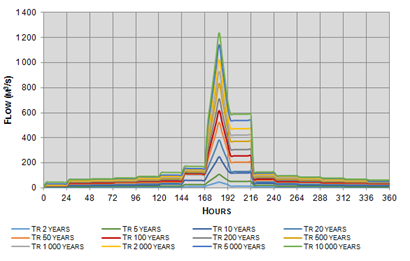Figure 1 Majorated design floods. La Boca Dam.

Optimal operation

Considering the average daily flows, as well as the characteristics of the reservoir presented in Table 2, the optimization of long-term operation policy was performed.17–19 Runoff matrix for the reservoir analytical simulation was built in to fortnightly intervals. To distribute the annual average volume in to intervals throughout the year (considering the flood and dry seasons), six monthly groups were defined, which are conformed as showed in Table 3. Considering the useful capacity of the dam (39.00 hm3), the water volume has been distributed in 50 states by 0.7898 hm3 each one. The absolute frequencies of runoff volumes, for each state and group of months, are defined in Table 3; the relative frequencies were obtained dividing the absolute frequencies between the total numbers of years of the sample, this analysis has been considered as an approach to the probabilities associated with incomes of runoff matrix. Plotting the relative frequencies against the volume interval, discontinuities appeared, so they were smoothed by distributing the volume maintaining the original shape form, forcing the sum to be equal to 1. From smoothed relative frequencies, it was developed the reservoir optimal operation policy, by stochastic dynamic programming.20 In Figure 2 the annual summary of levels obtained from the simulation of the optimal operation policy are presented, in particular, the average biweekly levels in the reservoir that are compared with historical levels. As can be seen in Figure 2, the operation policy obtained from the simulation preserves the levels above the historical average regime (increasing the generation), without reaching the maximum level of operation (NWL). Based on the levels obtained in the simulation, the relative frequencies histogram of elevations for the months from August to November was elaborated (considered as the flood season). As a result, Figure 3 presents these relative frequencies for class intervals of 1.5 m (the last interval to be considered is going to be the NWL, 448.50 m).

 Basin area 266,00 km2 Minimum annual runoff 20,08 hm3 Average annual runoff 70,71 hm3 Maximum annual runoff 213,24 hm3 Design flow (Tr 10 000 years) – Spillway capacity 2 250,00 m3/s Silt level 425,74 m NWL 448,05 m MWL 449,20 m Freeboard 0,84 m Silt level capacity 1,62 hm3 NWL capacity 39,49 hm3 Regulation capacity (NWL – MWL) 3,17 hm3 Total Capacity 42,66 hm3 Area at NWL 465,75 ha Area at MWL 498,36 ha

Table 2 General characteristics

 Group Month 1 January + February + March + April 2 May + June + July 3 August 4 September 5 October 6 November + December

Table 3 Monthly groups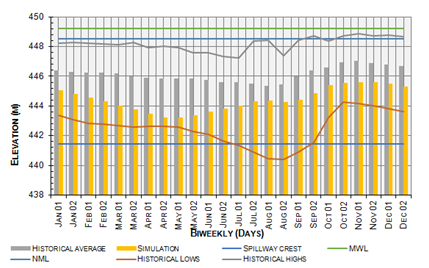Figure 2 Optimal operation vs historical levels.

Flood routing

Flood routing simulation was analyzed considering different initial water levels in the reservoir, in order to obtain the maximum discharge flow magnitude. "Reservoir flood routing” Marengo et al.21 program was used to analyze flood routing simulation. For this analysis two scenarios were considered:

1. Starting simulation at NWL (448.50 m),
2. Initial condition corresponding to the most frequently elevations obtained from the reservoir analytical simulation (Figure 3).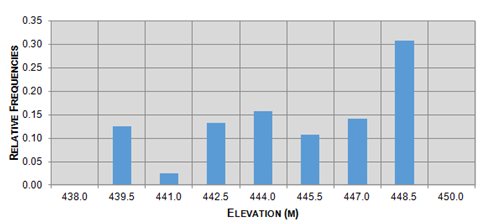Figure 3 Frequently average elevations histogram.

The income flows into the dam associated to different return periods are presented in Figure 1, (2, 5, 10, 20, 50, 100, 200, 500, 1 000, 2 000, 5 000 and 10 000 years).22

Stage 1 - Initial Level 448.50 m (NWL)

From the flood routing simulation on Figure 4, the results for a 10 000 years return period are shown. Figure 4, represents that for floods up to 10 000 years return period, the highest elevation obtained from analysis, 448,71 m, would be 0,49 m below MWL (449.20 m), so there is no danger for exceeding the maximum water level in the dam (MWL). The described analysis was performed in the same way for each return period associated to different recurrence intervals. Figure 5 represents analysis results trending, return periods (years) are plotted in relation to the maximum reached elevation in the reservoir.23Figure 4 Flood routing. Initial elevation h = 448.50 m. Tr = 10 000 years.

Scenario 2 - Frequently average levels in the reservoir

The analysis was elaborated starting from several initial levels in the reservoir. For this purpose, the level histogram (Figure 3) obtained from the reservoir optimal operation policy was used, resulting seven more frequent levels in the reservoir (Table 4), whose frequencies correspond to the Probabilities of occurrence to be analyzed. With initial levels defined in Table 4 and design floods presented in Figure 3, routing through the reservoir is performed as described below.24–26

 Intervals @ 1,5 m Absolute Frequencies Relative Frequencies 438,0 439,5 15,00 0,125 439,5 441,0 3,00 0,025 441,0 442,5 16,00 0,130 442,5 444,0 19,00 0,160 444,0 445,5 13,00 0,110 445,5 447,0 17,00 0,140 447,0 448,5 37,00 0,310 Total 120 1

Table 4 Reservoir levels frequencies

Initial level 439.5 m

Routing trough the reservoir from initial elevation at 439,50 m, for return periods from 2 to 10 000 years were analyzed, determining its maximum discharge flow rate, maximum volume in the reservoir and maximum elevation reached. The exceedance probabilities of maximum discharge flows considering values from 150 to 1 350 m3 /s, each 150 m3/s, were estimated by a linear interpolation between the results using the reduced variable , as shown in Figure 6. The described procedure was applied the same way for the next initial levels: 441, 442.5, 444, 445.5, 447 and 448.5 m (NWL).27–30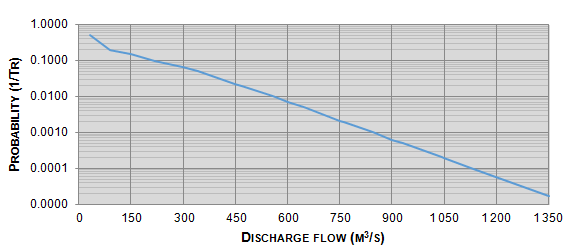Figure 6 Curve Maximum discharge rate vs Exceedance Probability - Initial level 439.50 m.

Overview results for different initial levels

Figure 7 shows the graphical analysis of the probability distributions of maximum discharge flows for the different initial levels studied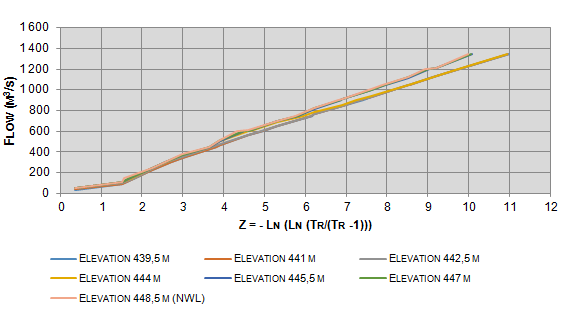Figure 7 Overview of the flows associated to different initial levels.

Joint exceedance probability analysis

On scenario number two, flood routing for different initial levels described are presented. The joint exceedance probability $\left({P}_{T}\right)$  was determined, with the exceedance probabilities obtained for each maximum flow discharge rate and the probability of occurrence associated to each initial lift ${P}_{Elev}$ .31 So, for each flow between 150 and 1 350 m3/s, it was performed the sum of the product of the probability associated with each baseline $\left({P}_{Elev}\right)$ multiplied by the exceedance probability the corresponding flow $\left({P}_{Q/Elev}\right)$ , it means:

${P}_{Ti}={\sum }_{k=439,5}^{448,5}{P}_{Q}{}_{i/k}{P}_{Elev}{}_{k}$ (7)

Where ${P}_{Ti}$ is the joint exceedance probability associated to the maximum discharge flow rate ; ${P}_{Elev}{}_{k}$ is the probability that the flood routing begins in $k$  elevation, and ${P}_{Q}{}_{i/k}$ is the probability that the maximum discharge flow rate be greater than or equal to given the initial elevation k. In Table 5, the joint exceedance probabilities obtained for each analyzed flows are presented: From the analysis, the procedure presented in Table 5 was performed for flows between 150 and 1 350 m3/s, at each 150 m3/s. Figure 8 presents the trend for joint exceedance probability associated with each maximum discharge flow rate, compared with the one obtained considering at the NWL.32–34

 Initial level Flow (m3/s) 150 m Tr (years) PQ PELEV PQ PELEv 439.5 6.67 0.15 0.125 0.01875 441 6.67 0.15 0.025 0.00375 442.5 6.67 0.15 0.133333 0.02 444 6.25 0.16 0.158333 0.025333 445.5 6.06 0.165 0.108333 0.017875 447 5.88 0.17 0.141667 0.024083 448.5 5.26 0.19 0.308333 0.058583 PT 150 0.168375

Table 5 Joint exceedance probability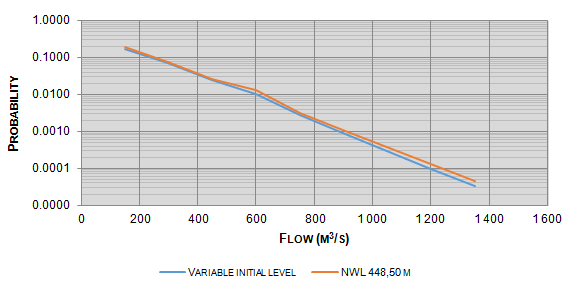Figure 8 Joint exceedance probabilities summary. La Boca Dam.

Hydraulic routing through the river

Considering maximum discharge flow, flood routing simulation through the river was performed. For this, the digital elevation model of the ASTER GDEM (2014) (ASTER Global Digital Elevation Model) page was used, with a resolution of 20 meters in the vertical plane and 30 meters in the horizontal plan, analyzing the area between the spillway of La Boca Dam, and the reservoir of Marte R. Gomez Dam, located downstream. This area was delimited using the Global Mapper ® platform (2014). The digital elevation model was exported to HEC-RAS ® program (2014) in order to carry out the simulation of the flood along the river, considering the different flows analyzed, as well as the physiographic characteristics of the river. The results obtained from the HEC RAS simulation were incorporated into the LAMINA ® program (2014), which determines the flooded area or surface from the water levels by each section of the river.35

# Allocation costs results

Based on costs per affected hectare at Chicoasén II Hydroelectric Project (90,090 Mexican pesos in 2014), it was considered to use a slight increase in the average inflation rate of 1% by 2015 (as the same way that El Chuchillo and Cerro Prieto dams), corresponding to a cost per Hectare of affectation of about 90 900 Mexican pesos; this cost was multiplied by the affected areas, determining the costs associated to different exceedance probabilities (Figure 9). The expected cost is determined as the area under the curves in Figure 10. The result is shown in Table 6, in which both scenarios are compared.36Figure 9 Costs associated to different exceedance probabilities.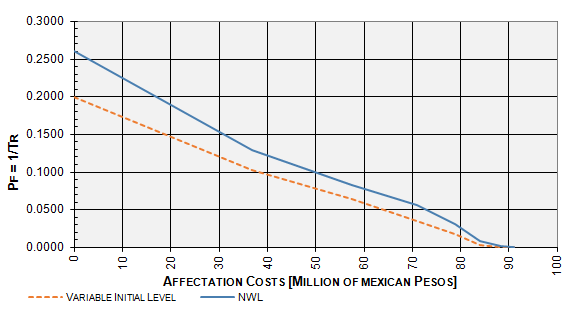Figure 10 Costs associated to different exceedance probabilities. El Cuchillo Dam.

 Analysis Millions of Mexican Pesos Variable initial level 4,76 NWL 5,20

Table 6 Total expected cost

# Comparison and results discussion

La Boca Dam spillway´s original design flood (Tr 10 000 years) corresponds to a maximum outflow of 2 250 m3/ s, on the other hand, from the hydrometric update a maximum inflow of 1 236 m3/s was obtained, leaving 1 014 m3/s at the maximum discharge capacity compared to original design. Updated flood routing simulation associated to a return period of 10 000 years by the reservoir, considering as an initial condition the elevation of the NWL (448.50 m), Figure 11 defines the maximum elevation in the reservoir is 448.71 m, this is 0.49 m below the MWL (449.20 m). The application of proposed methodology by Huerta & Domínguez1,2 reflects an average saving of 0.44 million pesos respect the conventional estimation method, however, comparing the results obtained with those of other reservoirs studied by Huerta and Domínguez, (El Cuchillo and Cerro Prieto Dams, Figures 10 and 11, respectively), the savings obtained from simulation are minimum. Table 7 shows the comparative summary of results obtained. As can be seen in the Table 7, the savings reflected for El Cuchillo (31%) and Cerro Prieto (54%) dams are highly significant compared to those obtained for La Boca Dam (9%). Therefore, it is possible to define that the methodology proposed by Huerta and Domínguez generates optimal results in reservoirs whose downstream border are not a plain area, as in the cases of El Cuchillo and Cerro Prieto dams. Due to the above, the savings obtained for the La Boca dam are minimal, however, the methodology optimizes the operation of the reservoir, guaranteeing the flow committed or demanded, safeguarding the reservoir elevation before an a extraordinary flood event.37,38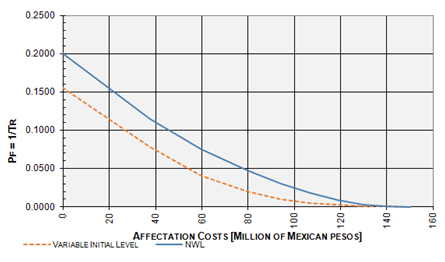Figure 11 Costs associated to different exceedance probabilities. Cerro Prieto Dam.

Analysis

El Cuchillo Dam

Cerro Prieto Dam

La Boca Dam

Millions of Mexican Pesos

Variable initial level

8.2

6.62

4.76

NWL

10.74

10.22

5.2

Saving

2.54

3.6

0.44

Saving percent

31%

54%

9%

Table 7 Total expected cost, summary

# Conclusion

Flooding risk downstream of a dam is traditionally assumed, considering that when the extraordinary event is happening, the reservoir is in the NWL, but in real operation conditions the reservoir levels differs of the NWL which implies in most common cases, an overestimation in affectations. The main goal of current investigation is analyze Huerta & Dominguez method in La Boca dam. The development of the method includes the simulation of design floods routing associated to different return periods (Dam and River flood routing), the definition of the long-term operation policy that optimizes an objective function and the estimation of damages. The optimization of the reservoir operation policy allows the preservation of levels in the dam above the average historical regime, increasing the useful volume in the reservoir to be used according to the committed demands (irrigation, drinking water supply and/or generation of electric energy), without exceeding the NWL. Considering the probability of occurrence ${P}_{Elev}$ of the levels in the reservoir obtained from the optimization of the operation policy, the safety of the reservoir was checked against any extraordinary discharge flow event $\left({P}_{Q}{}_{i/k}\right)$ . Accumulating the products of these probabilities by the relative frequencies associated with each initial level, and considering the damages for each discharge flow, the risk curve and the expected damage were obtained, which are compared those obtained with the traditional methodology that considers as initial level is the NWL. Results indicate that total risk obtained considering an initial level at the NWL (which is not a real condition) overestimates the costs, unlike the proposed approach, involving several initial levels in the reservoir and the corresponding probabilities. On the other hand, from the spillway operation review, several operation policies were defined, guaranteeing the optimal operation of the spillway for any extraordinary flood, without exceeding the MWL. The proposed methodology, considers the initial level in reservoir as a random variable that allows a punctual estimation of the damage caused by the risk to various design floods, optimizing operation in the reservoir for a given demand, such as: energy generation, irrigation, drinking water supply, regulation of floods, or a combination of these. The optimization generates great benefits in the design and operation of dams, for example: the savings obtained in the affectations, the correct dispatch of the demand, mitigating spills, implementation of guide curves and operation patterns. Present study confirms previous findings and contributes additional evidence that suggests that the methodology don’t generate optimal results in reservoirs whose downstream border is a plain area.

None.

# Conflict of interest

The author declares there is no conflict of interest.